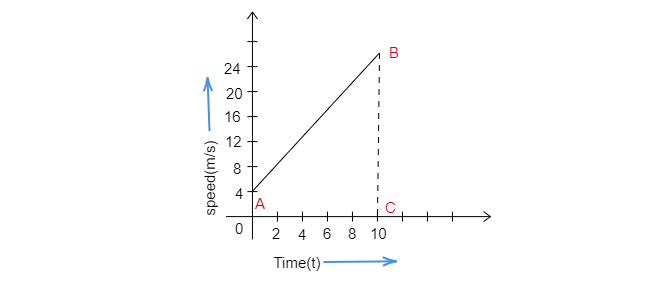Time(s)0246810Speed(m/s)4812162024
Draw the speed-time graph by choosing a convenient scale.
From this graph:
(i) Calculate the acceleration of the car.
(ii) Calculate the distance travelled by the car in 10 seconds."">

# A car is moving on a straight road with uniform acceleration. The speed of the car varies with time as follows:Time(s)0246810Speed(m/s)4812162024Draw the speed-time graph by choosing a convenient scale.From this graph:(i) Calculate the acceleration of the car.(ii) Calculate the distance travelled by the car in 10 seconds."

Speed-time graph of the moving car is as below:(i). Acceleration of the car $=$slope AB$=\frac{24-4}{10-0}$

$=\frac{20}{10}$

$=2\ m/s^2$

(ii). Distance traveled by car is equal to the area of trapezium OABC.

$=\frac{1}{2}\times(OA+BC)\times OC$

$=\frac{1}{2}\times(4+24)\times10$

$=\frac{1}{2}\times28\times10$

$=140\ m$

Updated on: 10-Oct-2022

56 Views